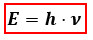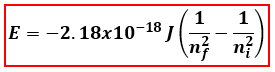# Problem: Calculate the frequency of the light emitted by a hydrogen atom during a transition of its electron from the n = 3 to n = 1 energy level, based on the Bohr theory. Use the equation En = -2.18 x 10 -18 J [(1/nf2)-(1/ni2)]a. 2.92 x 1015 s-1b. 3.56 x 1014 s-1c. 2.92 x 1014 s-1d. 1.17 x 1015 s-1

###### FREE Expert Solution

We will calculate the frequency of the light emitted by a hydrogen atom from the energy using the following equation:E = energy, J/photon
h = Planck' s constant = 6.626x10-34 J
s
ν = frequency

Let’s first calculate the energy of the hydrogen atom using the given Bohr Equation:ni = initial energy level
nf = final energy level###### Problem Details

Calculate the frequency of the light emitted by a hydrogen atom during a transition of its electron from the n = 3 to n = 1 energy level, based on the Bohr theory. Use the equation En = -2.18 x 10 -18 J [(1/nf2)-(1/ni2)]

a. 2.92 x 1015 s-1

b. 3.56 x 1014 s-1

c. 2.92 x 1014 s-1

d. 1.17 x 1015 s-1

What scientific concept do you need to know in order to solve this problem?

Our tutors have indicated that to solve this problem you will need to apply the Bohr and Balmer Equations concept. If you need more Bohr and Balmer Equations practice, you can also practice Bohr and Balmer Equations practice problems.

What is the difficulty of this problem?

Our tutors rated the difficulty ofCalculate the frequency of the light emitted by a hydrogen a...as high difficulty.

How long does this problem take to solve?

Our expert Chemistry tutor, Dasha took 3 minutes and 55 seconds to solve this problem. You can follow their steps in the video explanation above.

What professor is this problem relevant for?

Based on our data, we think this problem is relevant for Professor Sandusky's class at URI.